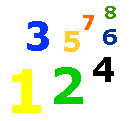# 1-41 Adding Two Numbers Up and Down

### Lesson List

Adding numbers up and down looks like this.

 2 + 5 7

It is the same as adding 2 + 5 = 7.

Look at the first number.  It is a 2.

Look at the second number.  It is a 5.

Begin with 2 and count forward five times. 2 » 3 » 4 » 5 » 6 » 7.

Practice adding numbers up and down.

Fill in the boxes.

 6 2 4 6 9 5 3 8 6 4 + 3 + 7 + 5 + 2 + 1 + 4 + 6 + 1 + 1 + 5 4 3 2 6 7 4 1 9 5 8 + 5 + 2 + 8 + 1 + 3 + 5 + 8 + 1 + 0 + 2 3 0 8 2 4 9 6 8 1 7 + 6 + 0 + 1 + 5 + 4 + 1 + 1 + 0 + 2 + 3